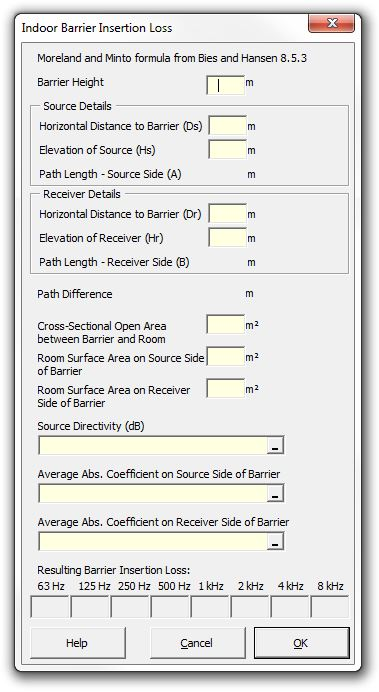### Strutt Help

Indoor Barrier Insertion Loss    1/1,1/3

Strutt|Building Acoustics|Indoor Barrier calculates the transmission loss through an indoor barrier, taking into account the reverberant sound field and reflections from other surfaces, and inserts the result into the active work sheet.Strutt implements the formula as:

IL = 10log_10(Q/(4 pi r^2)+4/(S_0 bar(alpha_0))) - 10log_10((QF)/(4 pi r^2)+(4 K_1 K_2)/(S(1-K_1 K_2)))
where:
IL is the barrier insertion loss
Q is the source directivity factor in the direction of the receiver (back-calculated from directivity index DI via DI=10log(Q)
r is the distance between source and receiver in the absence of the barrier
S_0 is the total room surface area
bar(alpha_0) is the mean root Sabine absorption coefficient
S is the open area between the barrier perimeter and the room walls and ceiling
F is the diffraction coefficient and is given by the following equation:

F = sum_i 1/(3+10N_i)

Where:
N_i is the Fresnel number for diffraction around the ith edge of the barrier and is given by the following equation:

N = +-(2/lamda)(A+B-d)

K_1 and K_2 are dimensionless numbers given by the following equation:

K_1 = S/(S+S_1 bar(alpha_1)), K_2 = S/(S+S_2 bar(alpha_2))

Where:
S_1 and S_2 are the total room surface areas on sides 1 and 2 respectively of the barrier
bar(alpha_1) and bar(alpha_2) are the mean Sabine Coefficients associated with areas S_1 and S_2 respectively

References:
Bies and Hansen, Engineering Noise Control, Second Edition, E & FN Spon, London 1996